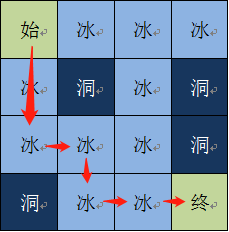IT课程

IT技术专业学习平台
IT人才专业服务提供商

# 人工智能强化学习07|动态规划：策略• Q值学习过程

Function 计算Q值(R, V)：

For s in S：

For a in A:

Return Q(s,a)• 高级智能——策略π格子名称：s1 V(s1) = 0 r(s1_右) = +1 q(s1_右) = 3 r(s1_下) = +1 q(s1_下) = 2 格子名称：s2 V(s2) = 2 r(s2_左) = -1 q(s2_左) = -1 r(s2_下) = +5 q(s2_下) = 10 格子名称：s3 V(s2) = 1 r(s2_上) = -1 q(s2_上) = -1 r(s2_右) = +5 q(s2_右) = 10 终点 V=5

 路线 Q值 Q值 S1 – S2 – 终点 3 10 S1 – S3 – 终点 2 10

• Try it yourself!(The End)

2018年8月29日 18:30